# Wedderburn-Mal'tsev theorem

Letbe a finite-dimensional associative algebra (cf. Associative rings and algebras) over a fieldwith radical, and let the quotient algebra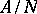be a separable algebra (for algebras over a field of characteristic zero this is always true). Thencan be decomposed (as a linear space) into a direct sum of the radicaland some semi-simple subalgebra: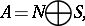and if there exists another decomposition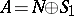, where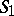is a semi-simple subalgebra, then there exists an automorphismof the algebrawhich mapsonto(the automorphismis inner, i.e. there exist elementssuch that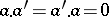andfor all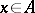, where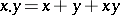). The existence of this decomposition was shown by J.H.M. Wedderburn  and the uniqueness, up to an automorphism of the semi-simple term, was proved by A.I. Mal'tsev . This theorem, together with Wedderburn's theorem (cf. Associative rings and algebras) on the structure of semi-simple algebras constitutes the central part of the classical theory of finite-dimensional algebras.